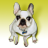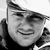Announcements
You can succeed best and quickest by helping others to succeed. Join the conversation.
cancel
Showing results for
Did you mean:Creator

## Set Analysis problem

Hi,

I would like to create a straight table based on the example data in the excel file. The condition is mentioned in the excel file. I need to calculate the sum of Amounts only for the red marked IDs and Type field.Thanks,

BC

Labels (1)
• ### Set Analysis

1 Solution

Accepted SolutionsMVP

Can I rephrase your condition to this?

Calculate the sum amount but exclude all amount for the IDs that is associated with type B.

If so then try this expression.

Sum({<ID=E({<Type={'B'}>}) [Aumount(\$)])

3 RepliesMVP

Can I rephrase your condition to this?

Calculate the sum amount but exclude all amount for the IDs that is associated with type B.

If so then try this expression.

Sum({<ID=E({<Type={'B'}>}) [Aumount(\$)])Creator
Author

Thank you so much Vegar!

-BCCreator

Sum({<Type={'A'}>}[Amount(\$)])Community Browser